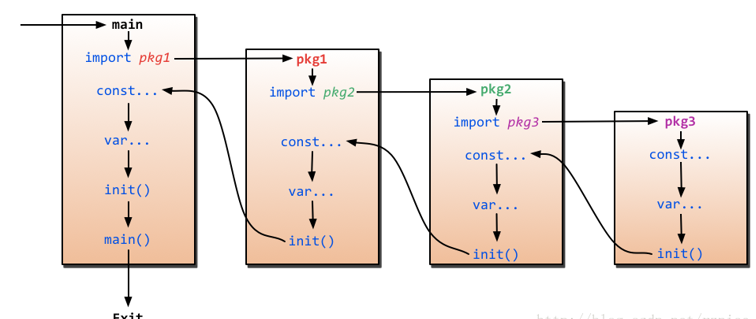# Go 流程和函数

2022-05-13 17:54 更新

## 流程控制

### if

`if`也许是各种编程语言中最常见的了，它的语法概括起来就是:如果满足条件就做某事，否则做另一件事。

Go里面`if`条件判断语句中不需要括号，如下代码所示

``````if x > 10 {
fmt.Println("x is greater than 10")
} else {
fmt.Println("x is less than 10")
}``````

Go的`if`还有一个强大的地方就是条件判断语句里面允许声明一个变量，这个变量的作用域只能在该条件逻辑块内，其他地方就不起作用了，如下所示

``````// 计算获取值x,然后根据x返回的大小，判断是否大于10。
if x := computedValue(); x > 10 {
fmt.Println("x is greater than 10")
} else {
fmt.Println("x is less than 10")
}

//这个地方如果这样调用就编译出错了，因为x是条件里面的变量
fmt.Println(x)``````

``````if integer == 3 {
fmt.Println("The integer is equal to 3")
} else if integer < 3 {
fmt.Println("The integer is less than 3")
} else {
fmt.Println("The integer is greater than 3")
}``````

### goto

Go有`goto`语句——请明智地使用它。用`goto`跳转到必须在当前函数内定义的标签。例如假设这样一个循环：

``````func myFunc() {
i := 0
Here:   //这行的第一个词，以冒号结束作为标签
println(i)
i++
goto Here   //跳转到Here去
}``````

### for

Go里面最强大的一个控制逻辑就是`for`，它即可以用来循环读取数据，又可以当作`while`来控制逻辑，还能迭代操作。它的语法如下：

``````for expression1; expression2; expression3 {
//...
}``````

`expression1``expression2``expression3`都是表达式，其中`expression1``expression3`是变量声明或者函数调用返回值之类的，`expression2`是用来条件判断，`expression1`在循环开始之前调用，`expression3`在每轮循环结束之时调用。

``````package main
import "fmt"

func main(){
sum := 0;
for index:=0; index < 10 ; index++ {
sum += index
}
fmt.Println("sum is equal to ", sum)
}
// 输出：sum is equal to 45``````

``````sum := 1
for ; sum < 1000;  {
sum += sum
}``````

``````sum := 1
for sum < 1000 {
sum += sum
}``````

``````for index := 10; index>0; index-- {
if index == 5{
break // 或者continue
}
fmt.Println(index)
}
// break打印出来10、9、8、7、6
// continue打印出来10、9、8、7、6、4、3、2、1``````

`break``continue`还可以跟着标号，用来跳到多重循环中的外层循环

`for`配合`range`可以用于读取`slice``map`的数据：

``````for k,v:=range map {
fmt.Println("map's key:",k)
fmt.Println("map's val:",v)
}``````

``````for _, v := range map{
fmt.Println("map's val:", v)
}``````

### switch

``````switch sExpr {
case expr1:
some instructions
case expr2:
some other instructions
case expr3:
some other instructions
default:
other code
}``````

`sExpr``expr1``expr2``expr3`的类型必须一致。Go的`switch`非常灵活，表达式不必是常量或整数，执行的过程从上至下，直到找到匹配项；而如果`switch`没有表达式，它会匹配`true`

``````i := 10
switch i {
case 1:
fmt.Println("i is equal to 1")
case 2, 3, 4:
fmt.Println("i is equal to 2, 3 or 4")
case 10:
fmt.Println("i is equal to 10")
default:
fmt.Println("All I know is that i is an integer")
}``````

``````integer := 6
switch integer {
case 4:
fmt.Println("The integer was <= 4")
fallthrough
case 5:
fmt.Println("The integer was <= 5")
fallthrough
case 6:
fmt.Println("The integer was <= 6")
fallthrough
case 7:
fmt.Println("The integer was <= 7")
fallthrough
case 8:
fmt.Println("The integer was <= 8")
fallthrough
default:
fmt.Println("default case")
}``````

``````The integer was <= 6
The integer was <= 7
The integer was <= 8
default case``````

## 函数

``````func funcName(input1 type1, input2 type2) (output1 type1, output2 type2) {
//这里是处理逻辑代码
//返回多个值
return value1, value2
}``````

• 关键字`func`用来声明一个函数`funcName`
• 函数可以有一个或者多个参数，每个参数后面带有类型，通过`,`分隔
• 函数可以返回多个值
• 上面返回值声明了两个变量`output1``output2`，如果你不想声明也可以，直接就两个类型
• 如果只有一个返回值且不声明返回值变量，那么你可以省略 包括返回值 的括号
• 如果没有返回值，那么就直接省略最后的返回信息
• 如果有返回值， 那么必须在函数的外层添加return语句

``````package main
import "fmt"

// 返回a、b中最大值.
func max(a, b int) int {
if a > b {
return a
}
return b
}

func main() {
x := 3
y := 4
z := 5

max_xy := max(x, y) //调用函数max(x, y)
max_xz := max(x, z) //调用函数max(x, z)

fmt.Printf("max(%d, %d) = %d\n", x, y, max_xy)
fmt.Printf("max(%d, %d) = %d\n", x, z, max_xz)
fmt.Printf("max(%d, %d) = %d\n", y, z, max(y,z)) // 也可在这直接调用它
}``````

### 多个返回值

Go语言比C更先进的特性，其中一点就是函数能够返回多个值。

``````package main
import "fmt"

//返回 A+B 和 A*B
func SumAndProduct(A, B int) (int, int) {
return A+B, A*B
}

func main() {
x := 3
y := 4

xPLUSy, xTIMESy := SumAndProduct(x, y)

fmt.Printf("%d + %d = %d\n", x, y, xPLUSy)
fmt.Printf("%d * %d = %d\n", x, y, xTIMESy)
}``````

``````func SumAndProduct(A, B int) (add int, Multiplied int) {
Multiplied = A*B
return
}``````

### 变参

Go函数支持变参。接受变参的函数是有着不定数量的参数的。为了做到这点，首先需要定义函数使其接受变参：

``func myfunc(arg ...int) {}``

`arg ...int`告诉Go这个函数接受不定数量的参数。注意，这些参数的类型全部是`int`。在函数体中，变量`arg`是一个`int``slice`

``````for _, n := range arg {
fmt.Printf("And the number is: %d\n", n)
}``````

### 传值与传指针

``````package main
import "fmt"

//简单的一个函数，实现了参数+1的操作
a = a+1 // 我们改变了a的值
return a //返回一个新值
}

func main() {
x := 3

fmt.Println("x = ", x)  // 应该输出 "x = 3"

fmt.Println("x+1 = ", x1) // 应该输出"x+1 = 4"
fmt.Println("x = ", x)    // 应该输出"x = 3"
}``````

``````package main
import "fmt"

//简单的一个函数，实现了参数+1的操作
func add1(a *int) int { // 请注意，
*a = *a+1 // 修改了a的值
return *a // 返回新值
}

func main() {
x := 3

fmt.Println("x = ", x)  // 应该输出 "x = 3"

fmt.Println("x+1 = ", x1) // 应该输出 "x+1 = 4"
fmt.Println("x = ", x)    // 应该输出 "x = 4"
}``````

• 传指针使得多个函数能操作同一个对象。
• 传指针比较轻量级 (8bytes),只是传内存地址，我们可以用指针传递体积大的结构体。如果用参数值传递的话, 在每次copy上面就会花费相对较多的系统开销（内存和时间）。所以当你要传递大的结构体的时候，用指针是一个明智的选择。
• Go语言中`channel``slice``map`这三种类型的实现机制类似指针，所以可以直接传递，而不用取地址后传递指针。（注：若函数需改变`slice`的长度，则仍需要取地址传递指针）

### defer

Go语言中有种不错的设计，即延迟（defer）语句，你可以在函数中添加多个defer语句。当函数执行到最后时，这些defer语句会按照逆序执行，最后该函数返回。特别是当你在进行一些打开资源的操作时，遇到错误需要提前返回，在返回前你需要关闭相应的资源，不然很容易造成资源泄露等问题。如下代码所示，我们一般写打开一个资源是这样操作的：

``````func ReadWrite() bool {
file.Open("file")
// 做一些工作
if failureX {
file.Close()
return false
}

if failureY {
file.Close()
return false
}

file.Close()
return true
}``````

``````func ReadWrite() bool {
file.Open("file")
defer file.Close()
if failureX {
return false
}
if failureY {
return false
}
return true
}``````

``````for i := 0; i < 5; i++ {
defer fmt.Printf("%d ", i)
}``````

### 函数作为值、类型

``type typeName func(input1 inputType1 , input2 inputType2 [, ...]) (result1 resultType1 [, ...])``

``````package main
import "fmt"

type testInt func(int) bool // 声明了一个函数类型

func isOdd(integer int) bool {
if integer%2 == 0 {
return false
}
return true
}

func isEven(integer int) bool {
if integer%2 == 0 {
return true
}
return false
}

// 声明的函数类型在这个地方当做了一个参数

func filter(slice []int, f testInt) []int {
var result []int
for _, value := range slice {
if f(value) {
result = append(result, value)
}
}
return result
}

func main(){
slice := []int {1, 2, 3, 4, 5, 7}
fmt.Println("slice = ", slice)
odd := filter(slice, isOdd)    // 函数当做值来传递了
fmt.Println("Odd elements of slice are: ", odd)
even := filter(slice, isEven)  // 函数当做值来传递了
fmt.Println("Even elements of slice are: ", even)
}``````

### Panic和Recover

Go没有像Java那样的异常机制，它不能抛出异常，而是使用了`panic``recover`机制。一定要记住，你应当把它作为最后的手段来使用，也就是说，你的代码中应当没有，或者很少有`panic`的东西。这是个强大的工具，请明智地使用它。那么，我们应该如何使用它呢？

Panic

Recover

``````var user = os.Getenv("USER")

func init() {
if user == "" {
panic("no value for \$USER")
}
}``````

``````func throwsPanic(f func()) (b bool) {
defer func() {
if x := recover(); x != nil {
b = true
}
}()
f() //执行函数f，如果f中出现了panic，那么就可以恢复回来
return
}``````

### `main`函数和`init`函数

Go里面有两个保留的函数：`init`函数（能够应用于所有的`package`）和`main`函数（只能应用于`package main`）。这两个函数在定义时不能有任何的参数和返回值。虽然一个`package`里面可以写任意多个`init`函数，但这无论是对于可读性还是以后的可维护性来说，我们都强烈建议用户在一个`package`中每个文件只写一个`init`函数。

Go程序会自动调用`init()``main()`，所以你不需要在任何地方调用这两个函数。每个`package`中的`init`函数都是可选的，但`package main`就必须包含一个`main`函数。App下载